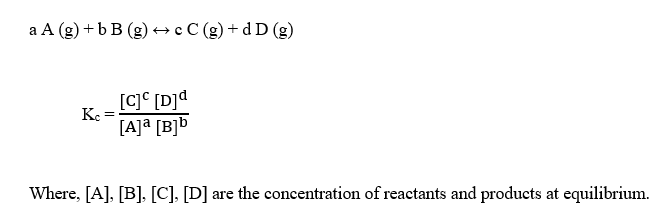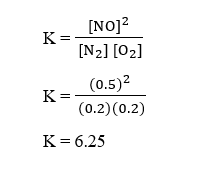# At equilibrium, the concentrations in this system were found to be [N2]=[O2]=0.200 M[N2]=[O2]=0.200 M and [NO]=0.500 M.[NO]=0.500 M.N2(g)+O2(g)−⇀↽−2NO(g)N2(g)+O2(g)↽−−⇀2NO(g)If more NONO is added, bringing its concentration to 0.800 M,0.800 M, what will the final concentration of NONO be after equilibrium is re‑established?

Question
223 views

At equilibrium, the concentrations in this system were found to be [N2]=[O2]=0.200 M[N2]=[O2]=0.200 M and [NO]=0.500 M.[NO]=0.500 M.

N2(g)+O2(g)−⇀↽−2NO(g)N2(g)+O2(g)↽−−⇀2NO(g)

If more NONO is added, bringing its concentration to 0.800 M,0.800 M, what will the final concentration of NONO be after equilibrium is re‑established?

check_circle

Step 1

The equilibrium constant, Kc for a reaction is given as :Step 2

Given,

Equilibrium concentration of N2 = 0.2 M

Equilibrium concentration of O2 = 0.2 M

Equilibrium concentration of NO = 0.5 M

The balanced reaction is given as :

N2 (g) + O2 (g) ↔ 2 NO (g)    K = ?

The equilibrium constant, K can be calculated as :Step 3

More NO is added, bringing its concentration to 0.800 M.

Now, since NO is added to the equilibrium reaction, to re-establish the equilibrium, the reaction will now move in backward direction according to Le-Chatelier’s principle, so given reaction becomes :

2 NO (g) ↔ N2 (g) + O2 (g)     K’ = 1/K = 1/6.2...

### Want to see the full answer?

See Solution

#### Want to see this answer and more?

Solutions are written by subject experts who are available 24/7. Questions are typically answered within 1 hour.*

See Solution
*Response times may vary by subject and question.
Tagged in